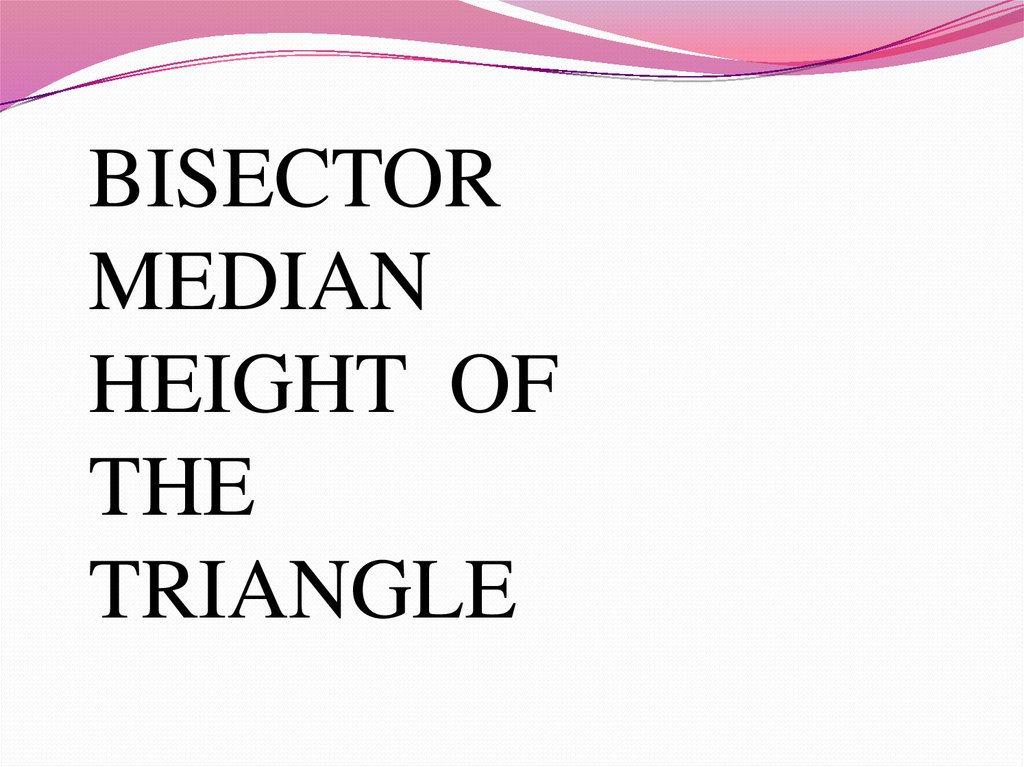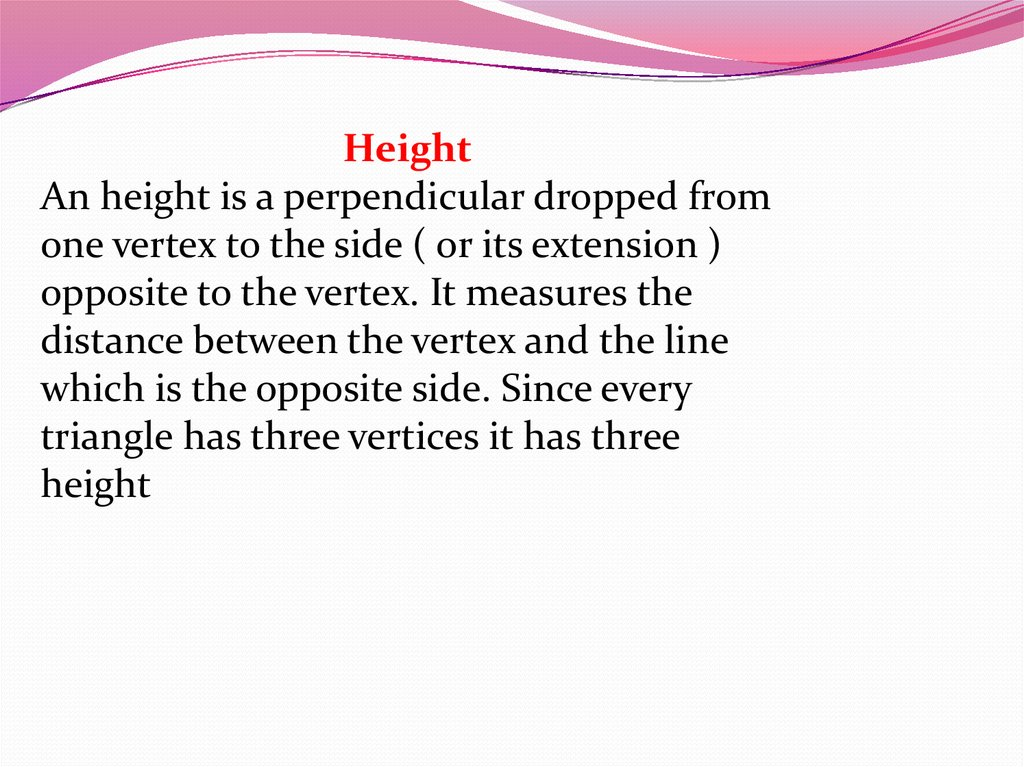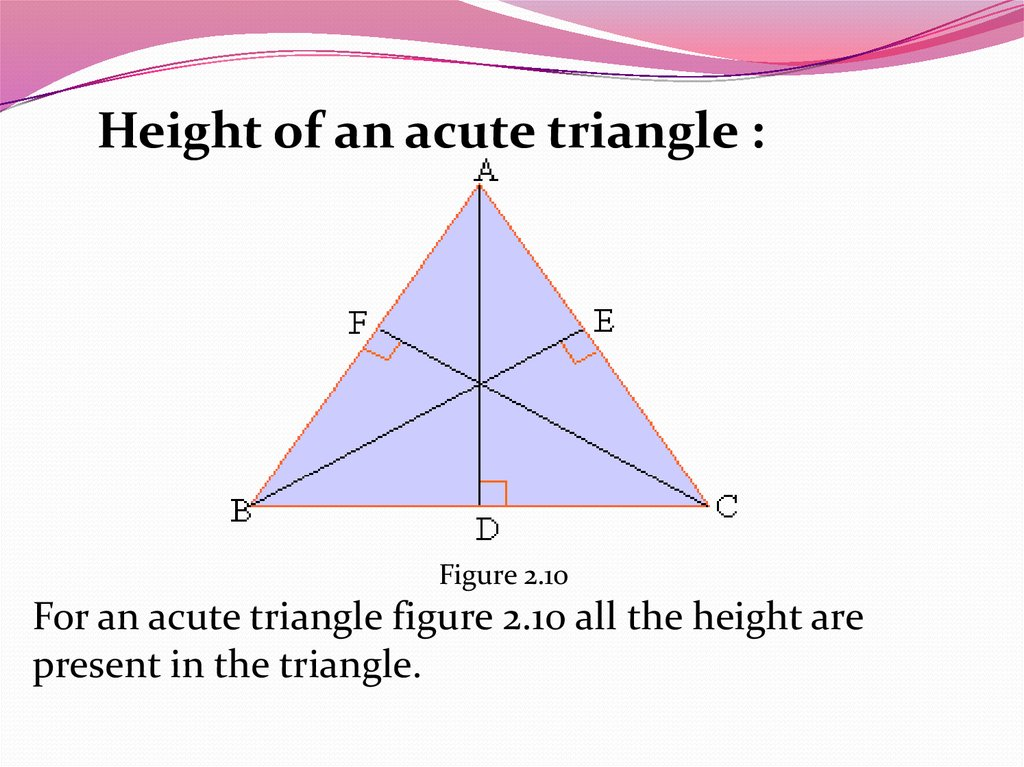# Bisector, median, height of the triangle

BISECTOR
MEDIAN
HEIGHT OF
THE
TRIANGLE

## 2.

Height
An height is a perpendicular dropped from
one vertex to the side ( or its extension )
opposite to the vertex. It measures the
distance between the vertex and the line
which is the opposite side. Since every
triangle has three vertices it has three
height

## 3.

Height of an acute triangle :
Figure 2.10
For an acute triangle figure 2.10 all the height are
present in the triangle.

## 4.

Height for a right triangle :
Figure 2.11
For a right triangle two of the height lie on the sides of the triangle, seg. AB is an
height from A on to seg. BC and seg. CB is an height from C on to seg.AB. Both of
them are on the sides of the triangle. The third height is seg. BD i.e.from B on to
AC. The intersection point of seg. AB, seg. BC and seg. BD is B. Thus for a right
triangle the three height intersect at the vertex of the right angle.

## 5.

Height for an obtuse triangle :
D ABC is an obtuse triangle. height from
A meets line containing seg.BC at D.
Therefore seg. AD is the height. Similarly
seg.CE is height on to AB and BF is the
height on to seg. AC. Of the three height,
only one is present inside the triangle.
The other two are on the extensions of
line containing the opposite side. These
three height meet at point P which is
outside the triangle.
Figure 2.12

## 6.

Median
A line segment from the vertex of a triangle to the midpoint of the
side opposite to it is called a median. Thus every triangle has three
medians. Figure 2.13 shows medians for acute right and obtuse
triangles.
Figure 2.13
All three medians always meet inside the triangle
irrespective of the type of triangle.

## 7. Angle Bisector A line segment from the vertex to the opposite side such that it bisects the angle at the vertex is called as

angle bisector. Thus every
triangle has three angle bisectors. Figure 2.14 shows angle bisectors
for acute right and obtuse triangles.
Figure 2.14